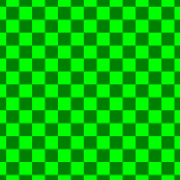xFace (geometry)EncyclopediaIn geometry
Geometry
Geometry arose as the field of knowledge dealing with spatial relationships. Geometry was one of the two fields of pre-modern mathematics, the other being the study of numbers ....

, a face of a polyhedron
Polyhedron
In elementary geometry a polyhedron is a geometric solid in three dimensions with flat faces and straight edges...

is any of the polygons that make up its boundaries. For example, any of the square
Square (geometry)
In geometry, a square is a regular quadrilateral. This means that it has four equal sides and four equal angles...

s that bound a cube
Cube
In geometry, a cube is a three-dimensional solid object bounded by six square faces, facets or sides, with three meeting at each vertex. The cube can also be called a regular hexahedron and is one of the five Platonic solids. It is a special kind of square prism, of rectangular parallelepiped and...

is a face of the cube. The suffix -hedron is derived from the Greek word hedra which means face.

Sometimes, in the case of a pyramid
Pyramid
A pyramid is a structure whose outer surfaces are triangular and converge at a single point. The base of a pyramid can be trilateral, quadrilateral, or any polygon shape, meaning that a pyramid has at least three triangular surfaces...

, the term face is understood to exclude the base.

The (two-dimensional) polygons that bound higher-dimensional polytopes are also commonly called faces. Formally, however, a face is any of the lower dimensional boundaries of the polytope, more specifically called an n-face.

## Formal definition

In convex geometry
Convex geometry
Convex geometry is the branch of geometry studying convex sets, mainly in Euclidean space.Convex sets occur naturally in many areas of mathematics: computational geometry, convex analysis, discrete geometry, functional analysis, geometry of numbers, integral geometry, linear programming,...

, a face of a polytope P is the intersection of any supporting hyperplane
Supporting hyperplane
Supporting hyperplane is a concept in geometry. A hyperplane divides a space into two half-spaces. A hyperplane is said to support a set S in Euclidean space \mathbb R^n if it meets both of the following:...

of P and P. From this definition it follows that the set of faces of a polytope includes the polytope itself and the empty set. For example, a polyhedron R3 is entirely on one hyperplane of R4. If R4 were spacetime, the hyperplane at t=0 supports and contains the entire polyhedron. Thus, by the formal definition, the polyhedron is a face of itself.

All of the following are the n-faces of a 4-dimensional polytope:
• 4-face - the 4-dimensional 4-polytope itself
• 3-face - any 3-dimensional cell
Cell (geometry)
In geometry, a cell is a three-dimensional element that is part of a higher-dimensional object.- In polytopes :A cell is a three-dimensional polyhedron element that is part of the boundary of a higher-dimensional polytope, such as a polychoron or honeycomb For example, a cubic honeycomb is made...

• 2-face - any 2-dimensional polygonal face (using the common definition of face)
• 1-face - any 1-dimensional edge
Edge (geometry)
In geometry, an edge is a one-dimensional line segment joining two adjacent zero-dimensional vertices in a polygon. Thus applied, an edge is a connector for a one-dimensional line segment and two zero-dimensional objects....

• 0-face - any 0-dimensional vertex
Vertex (geometry)
In geometry, a vertex is a special kind of point that describes the corners or intersections of geometric shapes.-Of an angle:...

• the empty set.

## Facets

If the polytope lies in m-dimensions, a face in the (m-1)-dimension is called a facet
Facet (mathematics)
A facet of a simplicial complex is a maximal simplex.In the general theory of polyhedra and polytopes, two conflicting meanings are currently jostling for acceptability:...

.
For example, a cell of a polychoron is a facet, a "face" of a polyhedron is a facet, an edge of a polygon is a facet, etc. A face in the (n-2)-dimension is called a ridge
Ridge (geometry)
In geometry, a ridge is an -dimensional element of an n-dimensional polytope. It is also sometimes called a subfacet for having one lower dimension than a facet.By dimension, this corresponds to:*a vertex of a polygon;...

.